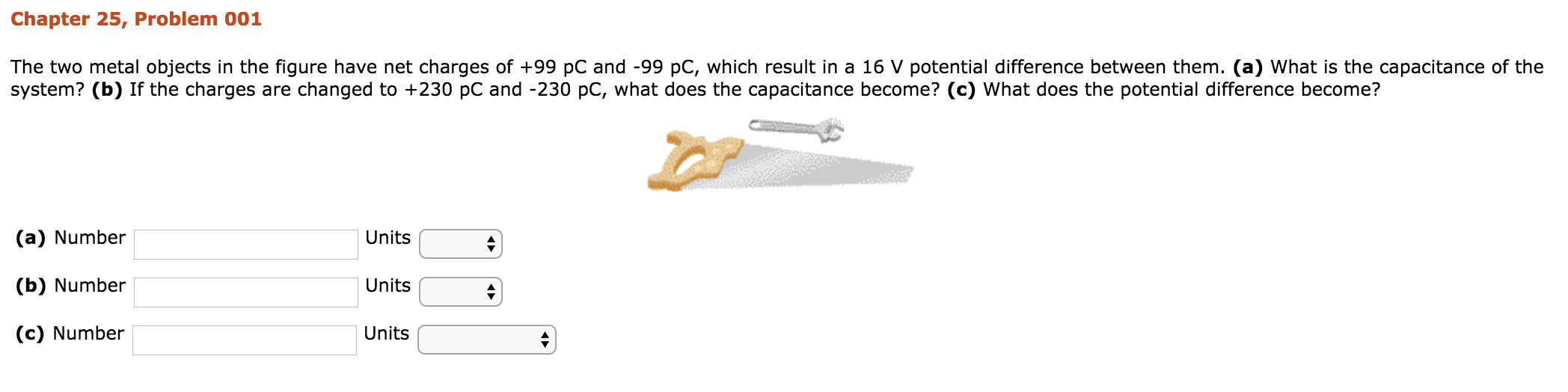# Chapter 25, Problem 001 The two metal objects in the figure have net charges of +99 pC and -99 pC, which result in a 16 V potential difference between them. (a) What is the capacitance of the system? (b) If the charges are changed to +230 pC and -230 pC, what does the capacitance become? (c) What does the potential difference become? (a) Number (b) Number (c) Number Units Units Units

Questionhelp_outlineImage TranscriptioncloseChapter 25, Problem 001 The two metal objects in the figure have net charges of +99 pC and -99 pC, which result in a 16 V potential difference between them. (a) What is the capacitance of the system? (b) If the charges are changed to +230 pC and -230 pC, what does the capacitance become? (c) What does the potential difference become? (a) Number (b) Number (c) Number Units Units Units fullscreen

### Want to see this answer and more?

Experts are waiting 24/7 to provide step-by-step solutions in as fast as 30 minutes!*